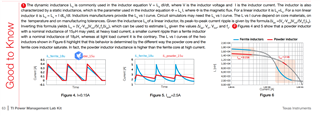If you have a related question, please click the "Ask a related question" button in the top right corner. The newly created question will be automatically linked to this question.

# TPS54160: Actual output ripple current

Part Number: TPS54160
Other Parts Discussed in Thread: PMLKBUCKEVM

Hi team,

We are testing the PMLKBUCKEVM, and we found that the actual output ripple current is much higher than the theoretical one, especially in the condition of Vin=24V, Vout=3.3V, fsw=250kHz. In that case, the theoretical output voltage is 633mA, but the actual one is 870mA. Could you pls help me to explain the reasons. Thanks a lot.

• HI Jerry:

pls provide detailed waveform with SW waveform , Vo ripple and Inductor current waveform as well as you EVM setup.

Thanks

Daniel Li

• Hi Daniel,

The EVM is setup as follows:

L = 18µH, Cout = 220µF, Cin = 10µF.

The following waveform of SW and output ripple current was tested by vin=6v,vout=3.3V with different load current. Moreover, the fsw, L, Cin and Cout is the same as what were mentioned above.Thanks a lot.

• HI

even indutor value mark is 18uH, there still a real inductor with a bit lower inductance. for this waveform, the real inductance is around 14uH.

have you read the experiment book which also decribed the dynamic inductance?• I haven't reached that point. Tanks for you answer. Have a good day!

• HI Jerry:

I will close this issue.

Thanks